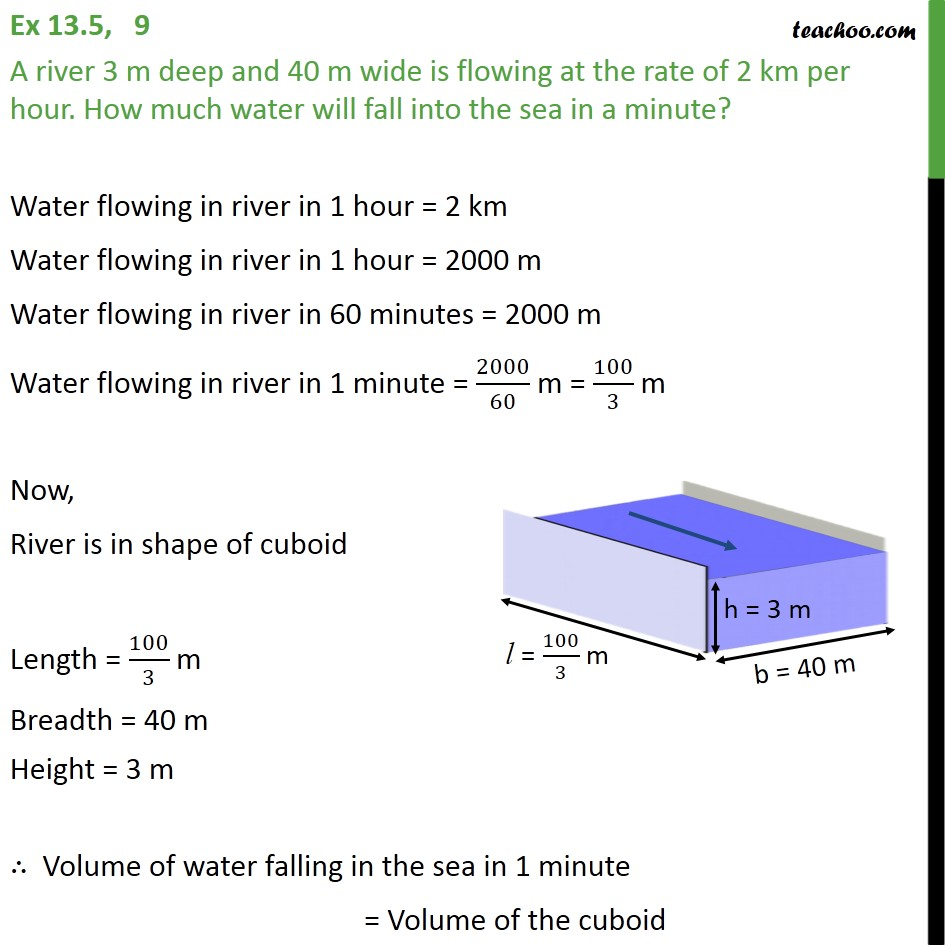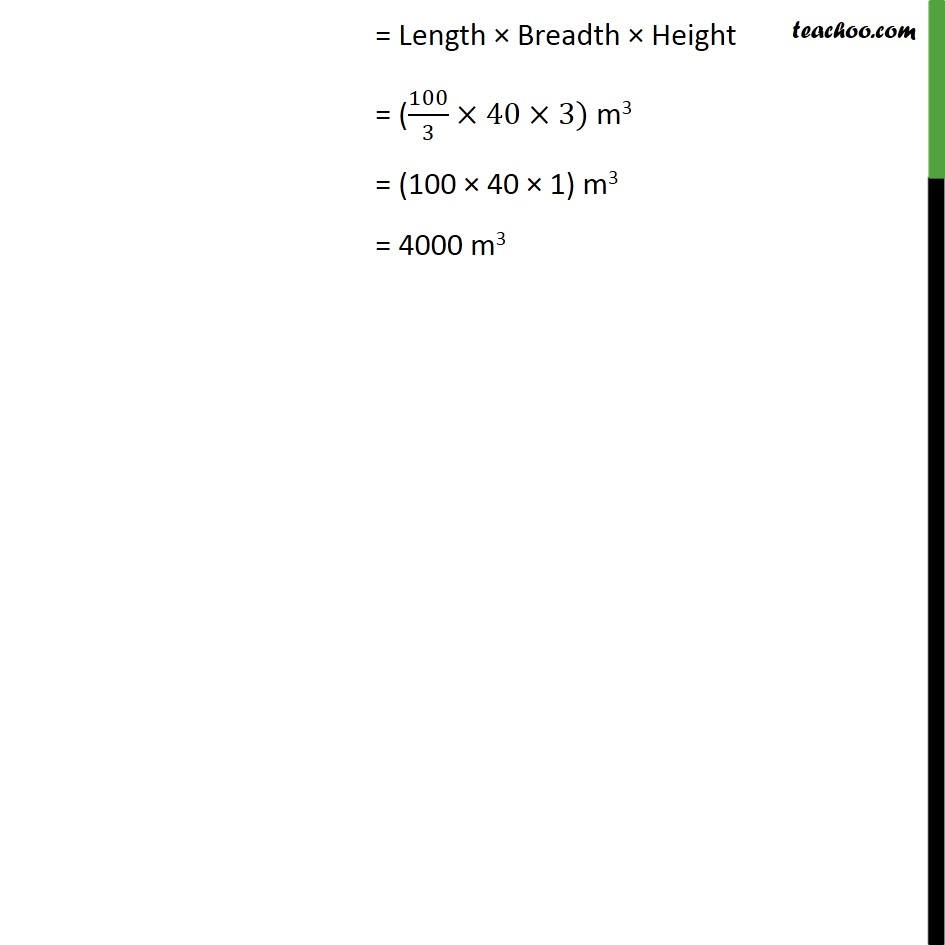Volume Of Cube/Cuboid

Chapter 11 Class 9 Surface Areas and Volumes
Concept wiseLearn in your speed, with individual attention - Teachoo Maths 1-on-1 Class

### Transcript

Question 9 A river 3 m deep and 40 m wide is flowing at the rate of 2 km per hour. How much water will fall into the sea in a minute? Water flowing in river in 1 hour = 2 km Water flowing in river in 1 hour = 2000 m Water flowing in river in 60 minutes = 2000 m Water flowing in river in 1 minute = 2000/60 m = 100/3 m Now, River is in shape of cuboid Length = 100/3 m Breadth = 40 m Height = 3 m ∴ Volume of water falling in the sea in 1 minute = Volume of the cuboid = Length × Breadth × Height = (100/3×40×3) m3 = (100 × 40 × 1) m3 = 4000 m3